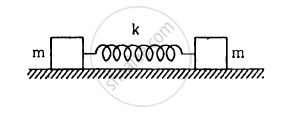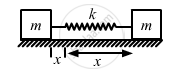Department of Pre-University Education, KarnatakaPUC Karnataka Science Class 11
Advertisement Remove all ads

# Consider the Situation Shown in Figure . Show that If the Blocks Are Displaced Slightly in Opposite Direction and Released, They Will Execute Simple Harmonic Motion. Calculate the Time Period. - Physics

Sum

Consider the situation shown in figure . Show that if the blocks are displaced slightly in opposite direction and released, they will execute simple harmonic motion. Calculate the time period.Advertisement Remove all ads

#### SolutionThe centre of mass of the system should not change during simple harmonic motion.
Therefore, if the block m on the left hand side moves towards right by distance x, the block on the right hand side should also move towards left by distance x. The total compression of the spring is 2x.
If v is the velocity of the block. Then
Using energy method, we can write:

$\frac{1}{2}k \left( 2x \right)^2 + \frac{1}{2}m v^2 + \frac{1}{2}m v^2 = C$

⇒ mv2 + 2kx2 = C
By taking the derivative of both sides with respect to t, we get:

$2mv\frac{dv}{dt} + 2k \times 2x\frac{dx}{dt} = 0$

$\text { Putting } v = \frac{dx}{dt}; \text { and } a = \frac{dv}{dt}\text { in above expression, we get }$

$ma + 2kx = 0$

$\Rightarrow - \frac{a}{x} = \frac{2k}{m} = \omega^2$

$\Rightarrow \omega = \sqrt{\frac{2k}{m}}$

$\Rightarrow \text { Time period }, T = 2\pi\sqrt{\left( \frac{m}{2k} \right)}$

Is there an error in this question or solution?
Advertisement Remove all ads

#### APPEARS IN

HC Verma Class 11, 12 Concepts of Physics 1
Chapter 12 Simple Harmonics Motion
Q 25 | Page 254
Advertisement Remove all ads
Advertisement Remove all ads
Share
Notifications

View all notifications

Forgot password?